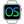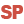Home |Sign in | EnglishHelpWLanguageWLanguage syntaxData typeAdvanced typesPointPresentationExampleWLanguage properties that can be used with the Point typeAssigning a Point variableAssigning an array of PointsComparison and serializationNotesSee alsoAdvanced typesBorderConstants for managing bordersFontPointProcedureUsing the advanced typesVariantMember
• WLanguage properties that can be used with the Point type
• Assigning a Point variable
• Assigning an array of Points
• Comparison and serialization
• Notes
WINDEVWEBDEVWINDEV MobileOthersPoint (Type of variable)
In french: Point
A Point variable is a structured type that is used to represent a position (X, Y) in 2 dimensions.Versions 24 and laterThis type of variable is now available for Java applications.New in version 24This type of variable is now available for Java applications.This type of variable is now available for Java applications.
Example
MyPoint is Point
MyPoint..X = 280
MyPoint..Y = 54
Syntax

Declaring and initializing a point

<Point name> is Point
<Point name>:
Name of the Point variable to declare.
Remarks

WLanguage properties that can be used with the Point type

The initial value of a point is set to (0,0).
The following properties can be used to handle a Point variable:
Property name Effect X X coordinate of the point. This coordinate corresponds to a signed 4-byte integer. Y Y coordinate of the point. This coordinate corresponds to a signed 4-byte integer.

Assigning a Point variable

A Point variable can be assigned with:
• another point.
For example:
MyPoint is Point
MyPoint..X = 280
MyPoint..Y = 54
MyPoint1 is Point = MyPoint
• an array of two values that can be converted into integer.
For example:
MyPoint is Point
MyPoint = [280, 54]

Assigning an array of Points

An array of Points can be assigned with:
• an array of values containing points.
For example:
ArrayOfPoints is array of Points
ArrayOfPoints = [MyPoint1,MyPoint2]
• an array of two values that can be converted into integer.
For example:
ArrayOfPoints is array of Points
ArrayOfPoints = [[1,2],[3,4]]

Comparison and serialization

• Comparison: The comparison operators = and <> are available.
• Serialization: The binary, XML and JSON serializations are available.
Caution: During an XML serialization, xmlAttribute cannot be used on a Point class member or on a Point structure member.

Notes

• A Point variable can be stored in a variant.
• A Point variable is never null:
• The "PointVariable = NULL" condition always returns False.
• The "PointVariable <> NULL" condition always returns True.
• You cannot reference a Point variable.
The "PointVariable2 <- PointVariable1" syntax triggers an error.
• You cannot force the copy of a Point variable.
The "PointVariable2 <= PointVariable1" syntax triggers an error.
• You have the ability to add and subtract two Point variables.
• The Point variables can be passed to the system APIs.
Minimum version required
• Version 21